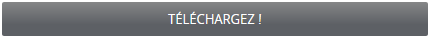# Learn Excel online Excel Basics for Account Reconciliation

## Learn Excel online Excel Basics for Account Reconciliation, tutoriel download PDF.

For example:
= 2 + 3 * 2 equals to 8
Not 10 because Excel calculates multiplication before addition.
You can control the order of calculation by using parentheses to group operations that should be performed first.
For example:
= (2 + 3) * 2
equals to 10
Entering functions
A basic function is a shortcut for a formula. Formulas and functions are a primary reason for using Excel, and it is essential that you start learning how easy they are to master.
Although there are some subtle differences between a formula and a function, many people use the words interchangeably.
The Excel Account Reconciliation worksheet you are using has a formula in column G that adds the values in each of the cells indicated in the formula. A formula is an equation that performs a calculation. You enter a formula in a cell at a location when some arithmetic is required.
When you press Enter, the results are displayed in the cell. A function is a built-in Excel formula. These built-in formulas cover many categories of commonly used mathematical, statistical, financial, and scientific operations.
An Excel function has syntax, which defines the necessary parts of the formula and the order of those parts. The syntax consists of an equal sign and the name of the function, followed by parentheses. Inside the parentheses, you place arguments. An argument is the information the function needs to complete its calculation, usually one or more values or cell addresses. Many functions use a cell range as an argument, a group of cells with a single address. A cell-range address includes the first cell, a colon, and the last cell.
The Components of a function include:
– Cell reference – indicates a cell’s location and provides instructions for how cell data is copied or used in calculations.
– Relative – cell value changes as the formula is copied
– Absolute – cell value remains static when copied to other locations
– Mixed – combination of an absolute and a relative cell
– Parentheses – control the Order of Operations
– Conditions or criteria tell the function how to calculate the results and what data to use.
……..

Introduction
Overview
Course objectives
Lesson 1 Getting Started
Overview
Starting the Program
Navigating in a Workbook
Selecting Cells Range in Workbook
Moving around in a range of cells – shortcut keys
Exploring the Ribbon
Using Dialog Box Launchers
Minimizing and Expanding the Ribbon
Exploring the Backstage View
Getting Help
Getting Help While You Work
Lesson 2: Basic Workbook Skills
Overview
Moving around a worksheet
Navigating with the mouse
Navigating with the keyboard
Selecting Ranges
Saving and updating workbooks
Saving a file
Saving Workbook in a different format
Summary Notes
Review Questions
Case Study – Create Worksheet from Actuals transactions
Lesson 3: Modifying a worksheet
Overview
Text and values
Overflow text and values
Editing text and values
AutoFill
Formatting Cells
Moving and copying data
Inserting and Deleting Rows, Columns and Cells
Freezing Panes in a Worksheet
Splitting a Worksheet window
Create a table
Checking Spelling in a Worksheet
Printing
Printing a portion of worksheet
End of Lesson Summary
Lesson 3 Review Questions
Case Study – Reformat Worksheet from Actuals transactions
Lesson 4: Using Formulas and Functions
Overview
Formulas
Operator precedence
Entering functions
Copy a Function
The Average Function
The MAX Function
The MIN Function
The MIN Function
Copying Formulas
Verifying a Formula Using Range Finder
Formatting a Worksheet
Copying a Range of Cells Across Columns to an Adjacent Range Using the Fill Handle
Changing the Workbook Theme
Formatting Dates and Centering Data in Cells
Accounting Number Format
Applying a Currency Style Format with a Floating Dollar Sign Using the Format Cells Dialog Box
Percent Style Format
Applying Conditional Formatting
…….